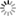##### Personal tools
You are here: Home Probability

# Probability

Randomness and uncertainty exist in our daily lives as well as in every discipline in science, engineering, and technology. Probability theory is a mathematical framework that allows us to describe and analyze random phenomena in the world around us. By random phenomena, we mean events or experiments whose outcomes we can't predict with certainty.

One way of thinking about "randomness" is that it's a way of expressing what we don't know. Perhaps if we knew more about the force I flipped the coin with, the initial orientation of the coin, the impact point between my finger and the coin, the turbulence in the air, the surface smoothness of the table the coin lands on, the material characteristics of the coin and the table, and so on, we would be able to definitively say whether the coin would come up heads or tails. However, in the absence of all that information, we cannot predict the outcome of the coin flip. When we say that something is random, we are saying that our knowledge about the outcome is limited, so we can't be certain what will happen.

Since the coin is fair, if we don't know anything about how it was flipped, the probability that it will come up heads is 50%, or 1212. What exactly do we mean by this? There are two common interpretations of the word "probability." One is in terms of relative frequency. In other words, if we flip the coin a very large number of times, it will come up heads about 1212 of the time. As the number of coin flips increases, the proportion that come up heads will tend to get closer and closer to 1212.

The beauty of probability theory is that it is applicable regardless of the interpretation of probability that we use (i.e., in terms of long-run frequency or degree of belief). Probability theory provides a solid framework to study random phenomena. It starts by assuming axioms of probability, and then building the entire theory using mathematical arguments.

[More to come ...]

##### Document Actions